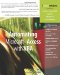# Using If...Then...Else

 < Day Day Up >

### Using If…Then…Else

The first branching statement that you should know about is the If statement. As you might guess from the word, the If statement executes code if something is true.

#### The Simple If Statement

To begin with, you can use If to control the execution of one or more statements. Here's an example:

` `

` Function IsSunday(dtmDayToCheck As Date) As Boolean   ' Returns true if the specified date is a Sunday   IsSunday = False   If DatePart("w", dtmDayToCheck) = 1 Then     IsSunday = True   End If End Function `

This function accepts a single date argument, and returns a Boolean value. If the DatePart function returns 1 when queried for the day of the week, the function returns True. Otherwise, it returns False, which is set as the function's default value by the first line of code.

Schematically, this simple form of the If statement looks like this:

` `

` If condition Then   statements End If `

The condition can be anything that returns a True or False value. If the condition returns True, all statements until the End If statement are executed in order. Otherwise, the entire block is skipped, and the first statement after the End If is executed next.

TIP

If a condition returns a numeric value, the value 0 is considered to be the same as False, and any other value is considered to be the same as True.

#### Creating More Complex Conditions

Conditions can use complex logic together with parentheses to group things if need be. For example, this function tests whether a date is Saturday or Sunday:

` `

` Function IsWeekend (dtmDayToCheck As Date) As Boolean   ' Returns true if the specified date is on a weekend   IsWeekend = False   If ((DatePart("w", dtmDayToCheck) = 1) Or _    (DatePart("w", dtmDayToCheck) = 7)) Then     IsWeekend = True   End If End Function `

Here the condition is spread across two lines. If the DatePart function returns True in either case, IsWeekend is set to True. Otherwise, it remains at the default value of False.

You'll frequently see one or more of the three logical keywords in a condition:

• The Or keyword is used when one or more conditions should be true. If you join two conditions with Or, the whole is true if either (or both) of the parts is true.

• The And keyword is used when all the conditions must be true. If you join two conditions with And, the whole is true only if all the parts are true.

• The Not keyword reverses true to false and vice versa.

Table 6.1 shows some examples of these logical keywords.

##### Table 6.1. Logical Keywords in Action

Condition

Explanation

(intDays = 4) Or (intWeeks = 3)

True if either intDays is 4, or intWeeks is 3, or both.

(intDays = 4) And (intWeeks = 3)

True if both intDays is 4 and intWeeks is 3.

Not (intDays = 4)

True if intDays has any value other than 4.

#### Adding the Else Statement

There are some optional parts to the If…End If structure. The first of these is the Else statement.

` `

` Function IsWeekday(dtmDayToCheck As Date) As Boolean   ' Returns true if the specified date is a weekday   If ((DatePart("w", dtmDayToCheck) = 1) Or _    (DatePart("s", dtmDayToCheck) = 7)) Then     IsWeekday = False   Else     IsWeekday = True   End If End Function `

Adding Else lets you return something if the condition is false. Schematically, it works like this:

` `

` If condition Then   statements1 Else   statements2 End If `

If the condition is True, the first set of statements is executed. Otherwise, the second set of statements is executed.

#### Using the ElseIf Statement

The other optional part to the If…End If structure is the ElseIf statement. Here's an example of this statement in action:

` `

` Function GetDayName(dtmDayToCheck) As String   ' Returns the day of the specified date   If DatePart("w", dtmDayToCheck) = 1 Then     GetDayName = "Sunday"   ElseIf DatePart("w", dtmDayToCheck) = 2 Then     GetDayName = "Monday"   ElseIf DatePart("w", dtmDayToCheck) = 3 Then     GetDayName = "Tuesday"   ElseIf DatePart("w", dtmDayToCheck) = 4 Then     GetDayName = "Wednesday"   ElseIf DatePart("w", dtmDayToCheck) = 5 Then     GetDayName = "Thursday"   ElseIf DatePart("w", dtmDayToCheck) = 6 Then     GetDayName = "Friday"   Else     GetDayName = "Saturday"   End If End Function `

Be sure to pass a valid date variable or a properly delimited date string using the # delimiting character. Otherwise, the function returns Saturday, regardless of the passed value. When VBA executes this function, it evaluates each of the conditions in turn, starting with the one following the If and continuing with each ElseIf. When it finds one that evaluates to True, it executes the corresponding statements and then skips to the End If statement. The main difference between Else and ElseIf is that you can state many different conditions instead of just one.

 < Day Day Up >Automating Microsoft Access with VBA
ISBN: 0789732440
EAN: 2147483647
Year: 2003
Pages: 186